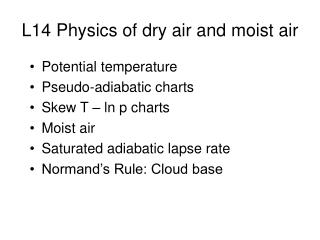DownloadDownload PresentationL14 Physics of dry air and moist air

# L14 Physics of dry air and moist air

Download Presentation## L14 Physics of dry air and moist air

- - - - - - - - - - - - - - - - - - - - - - - - - - - E N D - - - - - - - - - - - - - - - - - - - - - - - - - - -
##### Presentation Transcript

1. L14 Physics of dry air and moist air • Potential temperature • Pseudo-adiabatic charts • Skew T – ln p charts • Moist air • Saturated adiabatic lapse rate • Normand’s Rule: Cloud base

2. Potential Temperature (θ) • The potential temperature of an air parcel is its temperature when compressed (or expanded) adiabatically to surface pressure (p0) (defined as a standard pressure of 1000 hPa). • Again, start from the 1st Law of Thermodynamics, and make dq=0:

3. Ideal Gas Law (see Lecture 8) so: R is the specific gas constant for air R = 287 J kg-1 K-1 substitute in α:

4. Divide by RT: Integrate both sides, from the starting (p,T) tothe surface (p0,T0), noting cp/R is a constant: Remember integral of 1/x is the natural log of x:

5. R = 287 J kg-1 K-1 cp = 1004 J kg-1 K-1 Hence R/cp = 0.286 Integrating: Remember: Hence: or: Rearrange to give potential temperature, θ:

6. Happily, we can look at this graphically:e.g., the ‘Pseudo-adiabatic’ chart • So if you plot: p0.286 on y-axis,T on x-axis • For a constant θ, (p00.286/θ) is also a constant, so the graph yields a straight line with gradient given by (p00.286/θ), and passing through T=0 and p=0 Re-arrange:

7. Earth’satmosphere Pseudo-adiabatic chart Useful as now we can follow each line and determine graphically temperature at any pressure, assuming adiabatic expansion/compression y-axis is linear for p0.286 also linear for ln(p)

8. Earth’s atmosphere Pseudo-adiabatic chart Solves Poisson’s equation graphically! Disadvantage: Everything happens in small region of the chart… This can be overcome by skewing the temperature lines rather than plotting them straight up → The Skew T-ln p chart Earth’s atmosphere is never here

9. Skew T-ln pchartdifference to pseudo-adiabatic: ln(p) rather than p0.286T skewed • Examples: • Kuching in Malaysia • Valentia in Ireland. Vertical T Skew T

10. saturationmixingratio What are all the lines on the skew T-ln p chart? isobar isotherm dryadiabat saturated adiabat

11. Example: airplane air If an airplane at 250hPa takes air in at -51oC and adjusts it to cabin pressure (850 hPa), does the air have to be • Heated • Cooled to be comfortable?

12. Cabin pressure850 hPa Temperature ~43°C Followthe dry adiabatto 850 hPa

13. Moist air • See: L6 Humidity • Air contains some H2O molecules (water vapour) • Vapour pressure (e): partial pressure exerted by the gaseous water (hPa) • Mixing ratio (w): mass of water vapour / mass of dry air • Warmer air can accommodate more water molecules; the maximum for a given temperature is when the air is ‘saturated’ • For a given temperature, there is a:saturation vapour pressure (es)saturation mixing ratio (ws) • An air parcel can become saturated, e.g. by ascent and cooling • Once saturated, further cooling will result in condensation of liquid water: i.e. cloud droplets

14. evaporation condensation Mixing ratio w = mvapour/mdry [normally given units g/kg] At saturation: evaporation balances condensation Saturation mixing ratio ws = 0.622 es / p Relative Humidity = w/ws x 100% = e/es x 100%

15. Thermodynamics of saturated air • As long as air remains unsaturated, it will behave like ‘dry’ air • However, once saturated, the condensation of liquid water releases latent heat • This means that an ascending air parcel that becomes saturated will cool less than one that remains unsaturated • We can theoretically derive how much the cooling is modified (not done here, see Wallace & Hobbs p79-87 if interested), and define the ‘Saturated Adiabatic Lapse Rate’ (SALR) • The difference between the DALR and a SALR is largest for warmer air, as the water vapour content, and hence latent heat release are larger • Saturated adiabats are solid green lines on the skew T-ln p chart

16. Saturation mixing ratio Derived Using ideal gas law, and def. of saturation water vapour pressure (Clausius Clapeyron, Dr Essery)‏ Constant p: w increases with T Constant T: w increases with decreasing p

17. w = 6 g/kg ws Relative humidity: RH = 100*e/es ≈ 100*w/ws Dewpoint (Td): Temperature to which air must cool at constant pressure to be saturated Q: Air at 1000 hPa and 18oC has a mixing ratio of 6 g/kg. What is its relative humidity and dewpoint? RH=6/13*100=46% Dewpoint ~6.5oC

18. As unsaturated air lifts dry adiabatically, it will eventually saturate: Normand’s Rule

19. This level is the lifting condensation level

20. LCL = Cloud base

21. Let’s look at some real data

22. Albemarle, 00z Monday 17 Oct

23. Albemarle, 00z Tuesday 18 Oct

24. Summary • Potential temperature – the temperature of air compressed/expanded to 1000 hPa along a dry adiabat • Pseudo-adiabatic charts – graphically solve equations • Skew T – ln p charts – will use in labs • Moist air – releases latent heat at saturation point • Saturated adiabatic lapse rate – less than DALR – typically 6 K/km • Normand’s Rule: Can estimate cloud base height using surface temperature and moisture